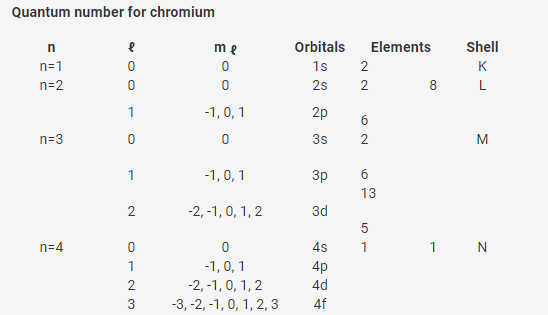# Write the electronic configuration of chromium and predict its number of subshells,

write the electronic configuration of chromium and predict its number of subshells, number of electrons in subshell having l=0, number of electrons having n+l value=3, number of electrons in the highest value of n.

The electron configuration for chromium is 1s22s22p63s23p64s23d4.

However, in the case of chromium there is one empty d sub orbital, one of the electrons from the 4s orbital will move up to the 3d orbital and make the the actual electron configuration 1s22s22p63s23p64s13d5.

Therefore,

Predicted electron configurations: Cr ( Z = 24): [Ar] 4 s23d4
Actual electron configurations: Cr ( Z = 24): [Ar] 4 s13d5(From the above table, we get,)
Predict its number of subshells
Ans: Chromium has 10 subshells viz - 1s, 2s, 2p, 3s, 3p, 3d, 4s, 4p, 4d, 4f

Number of electrons in subshell having l=0
Ans: There are 4 subshells and the first three sub shells (n=1, n=2 and n=3) has 2 electrons each and (n=4) has 1 electron.

Number of electrons having n + ℓ value=3
Ans: Total 8 electrons has n + ℓ value=3.

Number of electrons in highest value of n
Ans: Highest value is the last orbital (N orbital); n=4 i.e, 1 electron.